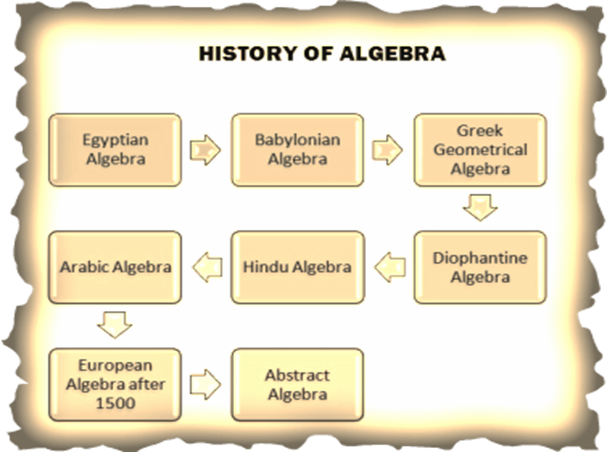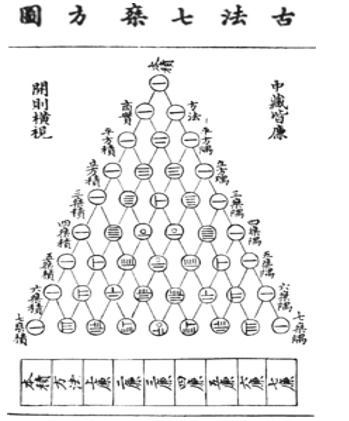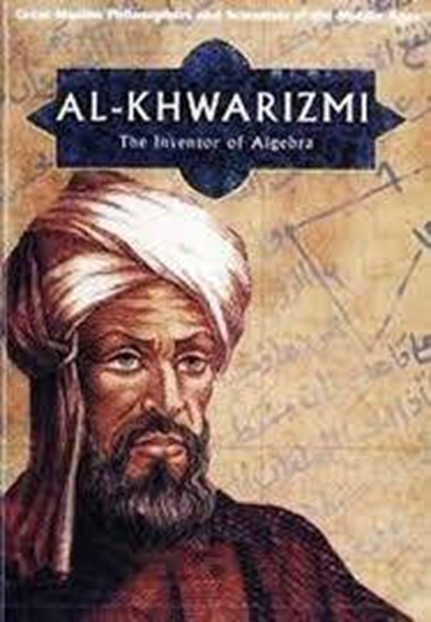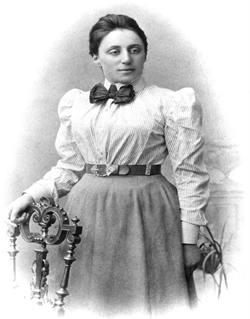Math & Beyond

# The History of Algebra

197 views

 1 Introduction 2 What is Algebra 3 History Of Algebra 3 Developmental Stages Of Algebra 4 Contributions of Different Countries 5 Contributions of Great Mathematicians 6 Summary 7 FAQs

27 October 2020

## Introduction

Mathematics as a subject can be broadly divided into three significant branches Arithmetic, Algebra, and Geometry. Algebra is considered to be one of the oldest components in the history of mathematics. Algebra deals with the study of symbols, exponentials, known and unknown variables, and equations. The History of Algebra has been dealt with in detail here.

## What Is Algebra?

The Number Theory, Geometry, and their analysis put together to make an extensive part of mathematics which is known as "Algebra". In other words, Algebra is a part of mathematics that deals with symbols and the rules to calculate those symbols.

## History Of Algebra

Muhammad ibn Musa al-Khwarizmi is known as the "Father of algebra". He was a Persian mathematician who wrote a book named Kitab Al Muhtasar fi Hisab Al Gabr Wa I Muqabala in the Arabic language, which was later translated into English as " The Compendious Book on Calculation by Completion and Balancing", from which the word ALGEBRA was derived. The book provides a systematic solution for linear and quadratic equations.

According to Al-Khwarizmi, the word algebra is described as 'reduction' and 'balancing' of subtracted terms that is a transposition to other sides of the equation (cancellation of like terms).

## Developmental Stages Of AlgebraThere are three critical developmental stages in "Symbolic Algebra" which is as follows :

### 1. Rhetorical Algebra

It was developed by ancient Babylonians where the equation was written in the form of words that remained up to the 16th century.

Example: x + 5 = 8, is written as " The thing plus five equal to eight"

### 2. Syncopated Algebra

Its expression first appeared in Diophantus Arithmetica (3rd century ) Brahmagupta's "Brahmagupta's sputa Siddhanta" (9th century), where few symbols were used, and subtraction was used only once in the equation.

### 3. Symbolic Algebra

At this stage, all the symbols were used in Algebra. Many Islamic mathematicians like Ibn Al-Banna and Al Qalasadi wrote in their books about Symbolic Algebra. Francois Viete fully developed it in the 16th century. Rene Descartes introduced a modern notation that can solve geometrical problems in terms of algebra known as Cartesian Geometry.

\begin{align}&x^2 + px = q\\[6pt]&x^2 = px + q\\[6pt]&x^2 + q = px \end{align}

In early algebra, Quadratic equations played an important role, where it is said to belong one among the above three equations.

# The Greeks and Vedic Indian Mathematicians developed two more stages of algebra which lie between rhetorical and syncopated stages known as Geometric Constructive stages.

## Contributions of different countries

### I. Babylon

Ancient Babylonians developed a rhetorical stage of algebra where equations were written in the form of words. They used linear interpolation to approximate intermediate values as they were not interested more in exact solutions. Plimpton 322 tablet, one of the most famous tablets designed around 1900 - 1600 BC gives the tables of "Pythagorean Triplets".

Following are the significant contributions :

• They developed flexible algebraic operations to eliminate fractions and factors by adding equals to equals and multiplying like quantities on both sides of the equation.
• They also knew simple forms of factoring, three-term quadratic equations with the positive term, and cubic equations

### II. Ancient Egypt

Ahmed, an Egyptian mathematician, wrote an Egyptian Papyrus in 1650 BC known as "The Rhind Papyrus" which is considered to be the most extensive ancient Egyptian Mathematical document in history. They mainly used linear equations.

The Rhind Papyrus contains problems of linear equation in the form of

x + xa = b and x + xa + bx = c where a, b and c are known terms and x is referred as " aha" or heap. The equations were solved by "method of false position" or "regular falsi" , in which a specific value is substituted to the left-hand side of the equation, and the obtained answer, after performing the required arithmetic operation is compared to the right-hand side of the equation.

### III. China

• ZHOUBI SUANGJING is one of the oldest Chinese Mathematical documents.

The following are the five major books by Chinese mathematicians in algebra.

 Nine Chapters on Mathematical Art It is one of the most influential books that gives solutions for determining and indeterminate simultaneous linear equations using both positive and negative numbers. In one of the problems, it has a solution for five unknowns in four equations. Sea-mirror of the Circle- measurement LI ZHI wrote this book where he solved equations with the highest degree as six by using Horner's method. Mathematical Treatise in nine section Ch'in Chiu-Shao, a wealthy governor and minister, invented the Chinese Remainder Theorem to solve simultaneous congruences. Magic Square In this book, the author Yang Hui formed a magic square or matrix by placing coefficients and constants to solve simultaneous linear equations. He worked with column reduction methods to get the solution. Precious mirror of the four elements Chu Shih-Chieh wrote this book in 1303 where unknown quantities in algebraic equations were represented as heaven, man, earth, and matter. Horner's method is used to solve the simultaneous equation with the highest degree of fourteen.Precious mirror of the four elements

### IV Greece

The Greek mathematician represented the sides of geometric objects, lines, and letters associated with them, which is called Geometric Algebra.

They invented "The application of areas " to obtain the solutions for equations solved in geometric algebra.

Following are few Greek mathematicians whose contribution are the milestone in the history of Algebra :

1. Thymaridas (c 400 BCE - 350 BCE) created a famous rule called

"Blooms Of Thymaridas" which states that

## Contributions of great mathematicians:

### 1. Muhammad ibn Musa al-Khwarizmi1. a Persian mathematician whose works have wide influence in mathematics, astronomy, and geography.
1. He wrote the book " The compendious book on calculation by completion and balancing" translated in Arabic as "Kitab Al muhtasar fi Hisab Al Gabr Wa I Muqabala" from which the word ALGEBRA was coined.
1. The book gives a systematic approach to solve linear and quadratic equations by reduction and balancing methods. Here are the steps used by him:
• Step 1 : reduction in which given equation is reduced to one of the following standard type,
 ax2 = bx Squares equal roots ax2 = c Squares equal number bx = c Roots equal number ax2 + bx = c Squares and roots equal numbers ax2 + c = bx Squares and numbers equal roots. bx + c = ax2 Roots and numbers equal squares.
• Step 2 : balancing by adding the same quantities to each side and removing negative roots, units and squares from equations.
 Example :

\begin{align}&x^2 + 16 = 9 + x\\[5pt]&x^2 + 16 - 9 = x\end{align}

Solution :  \begin{align}x^2 + 7 = x\end{align}

1. Al-Khwarizmi gave a unifying theory that created a new revolution in mathematical history where rational numbers, irrational numbers, geometrical magnitudes are treated as "Algebraic Objects ".

2. .Muhammad ibn Musa al-Khwarizmi contribution to Algebra made him to be addressed has "Father of Algebra"

### 2. Emmy NoetherAmalie Emmy Noether, a German mathematician, gave her contribution to Abstract Algebra. A famous theorem in Mathematical Physics has been titled in her name known as Noether theorem. She developed the Theories of Rings, fields and algebras. In physics Noether theorem explains the connection between symmetry and conservation laws.

Emmy Noether was born in a Jewish family in Franconian for Max Noether who was a mathematician. She studied mathematics at "University of Erlangen" She worked at the Mathematical Institute of Erlangen under the supervision of Paul Gordan in 1907.

In 1915 she got an invitation to join "the University of Gottingen" , a world renowned centre of mathematical research from David Hilbert, and Fliex Klien to join the mathematics department. She spent four years lecturing under David Hilbert's name. In 1919, she obtained "rank of privatdozent" after getting approval for her habilitation.

Noether's mathematical work is divided into three 'epochs'

• Contribution to Theories of algebraic invariants and number fields (1908 - 1919)
• Noether developed "theory of ideals in commutative rings " into a tool which had wide ranging applications that was published in her paper "Idealtheorie in Ringbereichen" (1920 - 1926)
• She published papers on noncommutative algebras and hypercomplex numbers and united the representation theory of groups with the theory of modules and ideals (1927 - 1935).

Pavel Alexandros, Albert Einstein, Jean Dieudonne, Herman Weyl, and Norbert Wiener described her as one of the most important women in the History of Mathematics. Her contribution to Abstract Algebra earned her the title " "

## Summary:

The History of Algebra almost started from the 9th century and the contributions of mathematics of different countries are infinite. Modern algebra is the evolution of all their works which has made it easy. The solution of quadratic equations with any number of exponentials can be obtained for both positive and negative integers by simple arithmetic analysis.

Written by Nethravati C, Cuemath Teacher

## Who is Muhammad ibn Musa al-Khwarizmi?

Muhammad ibn Musa al-Khwarizmi was a Muslim mathematician and astronomer who lived in Baghdad around the 9th century. He wrote a book called "kitab Al-Jabr" from which the word "ALGEBRA" derived.

## Who is the Father of Algebra?

Muhammad ibn Musa al-Khwarizmi is Known as "Father of algebra".

## Who is the Mother of Algebra?

Emmy Noether is Known as "The mother of modern algebra"

## What are the contributions of the Islamic world to Algebra?

• Arabic mathematicians were first to introduce algebra as an independent discipline in an elementary form.
• Emphasizes Hindu influence, emphasizes Mesopotamian or Persian- syriac influence, and emphasizes Greek influence are the three algebraic theories which have originated from ARABIC ALGEBRA.
• Al-Hassar, a mathematician from Morocco developed a special mathematical notation for fractions where the numerator and the denominator is separated by an horizontal bar.
• Omar Khayyam wrote a book on algebra which included 3rd degree algebraic equations. He provided both Arithmetic and geometric solutions for the quadratic equations.
• Al karkhi successor of Abu Al Wafa al buzjani was the first to discover the solutions for equations of the form . He is the first person to replace the geometrical operations with the arithmetic operations which are the core of algebra.

## When was algebra invented?

Muhammad ibn Musa al-Khwarizmi, a Muslim mathematician wrote a book in 9th century named "Kitab Al-Jabr" from which the word "ALGEBRA" derived. So algebra was invented in the 9th century.

Related Articles
Award-winning math curriculum, FREE for a year